# Conic sections questions and answers

Recent questions in Conic sectionsTolnaio 2021-08-11 Answered

### Find the equation of the graph for each conic in general form. Identify the conic, the center, the vertex, the co-vertex, the focus (foci), major axis, minor axis, ${a}^{2}$, ${b}^{2}$, and ${c}^{2}$. For hyperbola,find the asymtotes.Sketch the graph $16{x}^{2}-4{y}^{2}-64=0$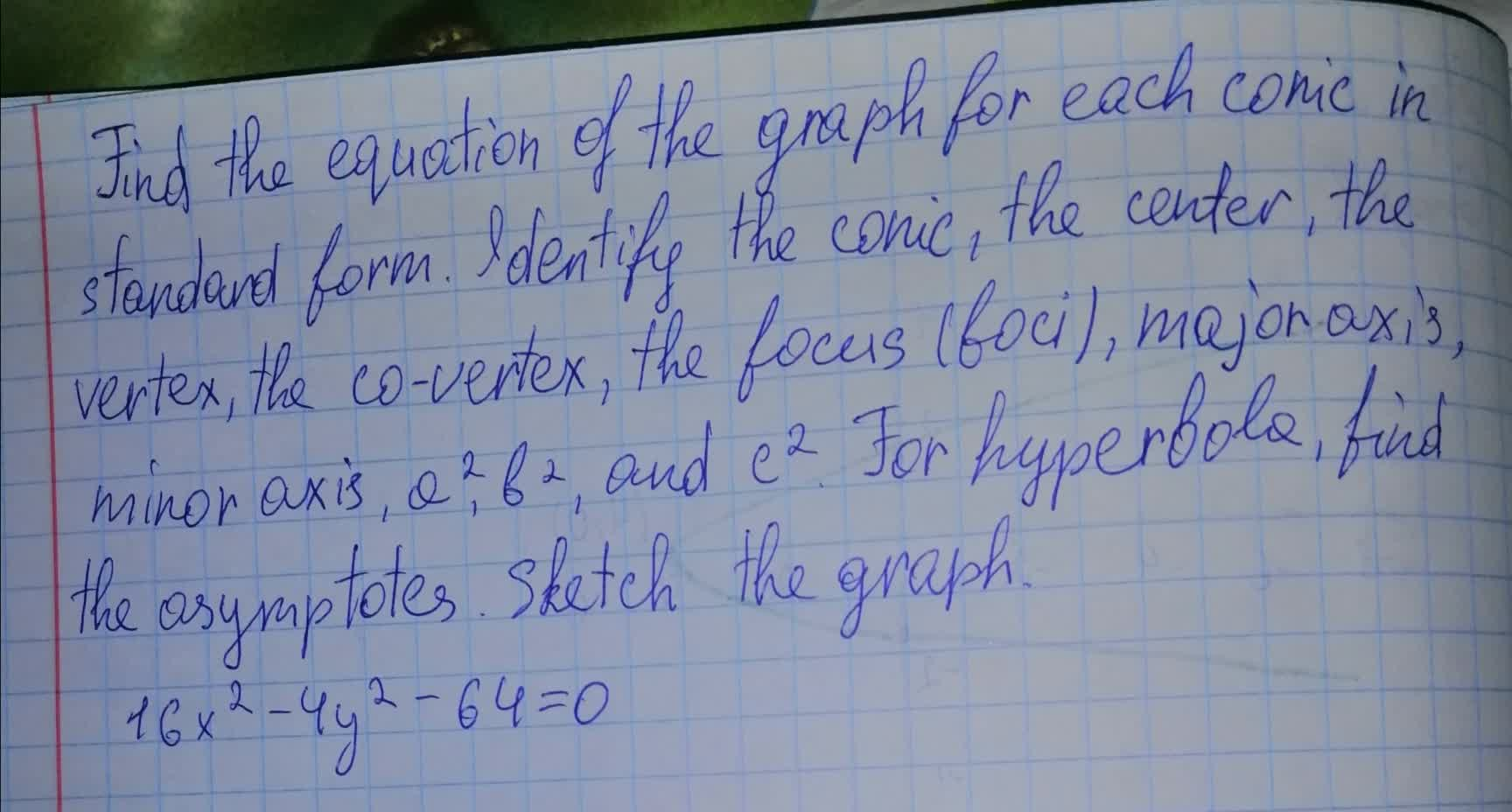tabita57i 2021-08-11 Answered

### Find the vertices and foci of the conic section. $\frac{{x}^{2}}{4}-\frac{{y}^{2}}{9}=36$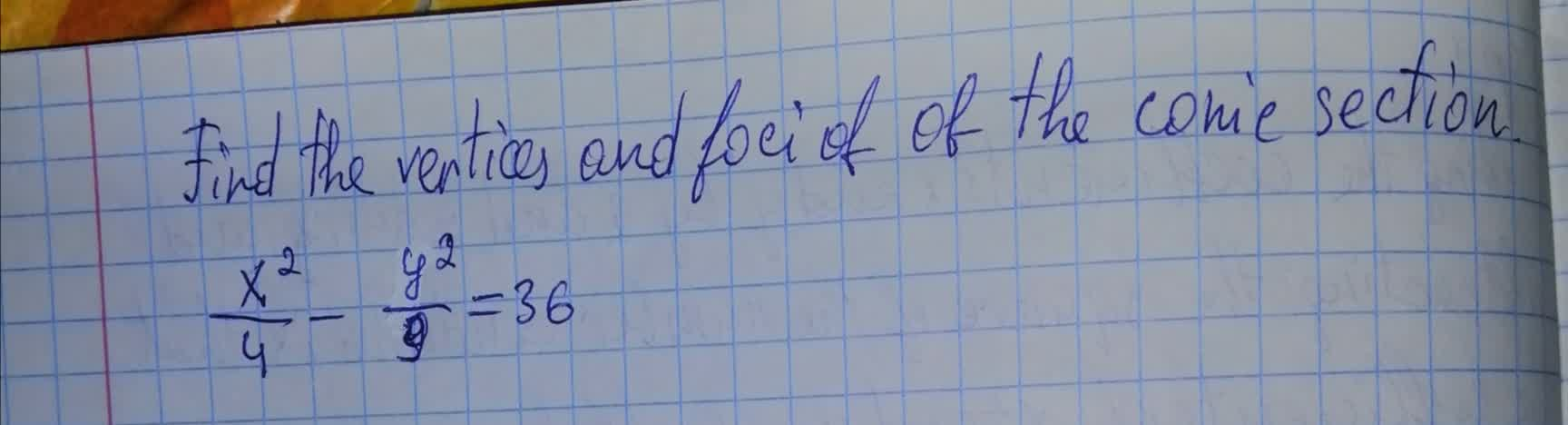Wierzycaz 2021-08-11 Answered

### Find the equation of the graph for each conic in standard form. Identify the conic, the center, the vertex, the co-vertex, the focus (foci), major axis, minor axis, ${a}^{2},{b}^{2}$, and ${c}^{2}$?. For hyperbola, find the asymptotes. Sketch the graph. $9{x}^{2}+25{y}^{2}+54x-50y-119=0$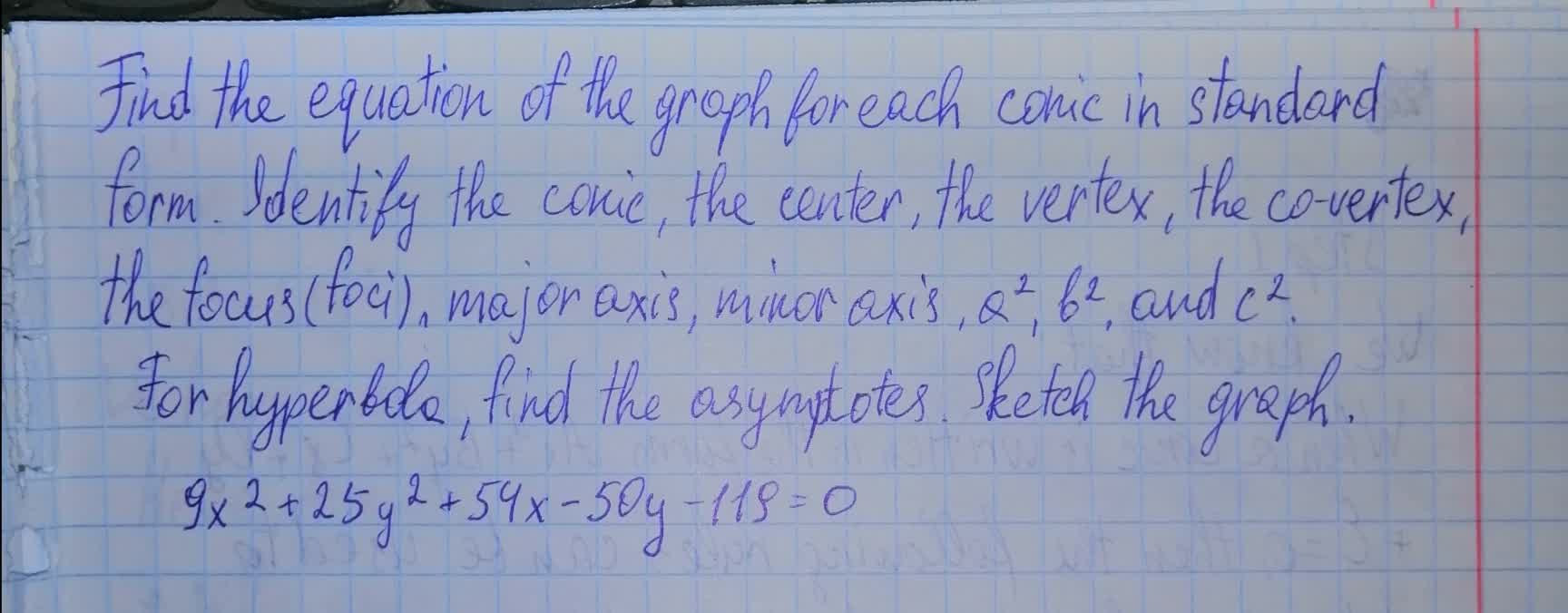pancha3 2021-08-10 Answered

### Show that the graph of an equation of the form $A{x}^{2}+C{y}^{2}+Dx+Ey+F=0,A\ne 0,C\ne 0$ where A and C are of opposite sign, (a) is a hyperbola if $\frac{{D}^{2}}{4A}+\frac{{E}^{2}}{4C}-F\ne 0$ (b) is two intersecting lines if $\frac{{D}^{2}}{4A}+\frac{{E}^{2}}{4C}-F=0$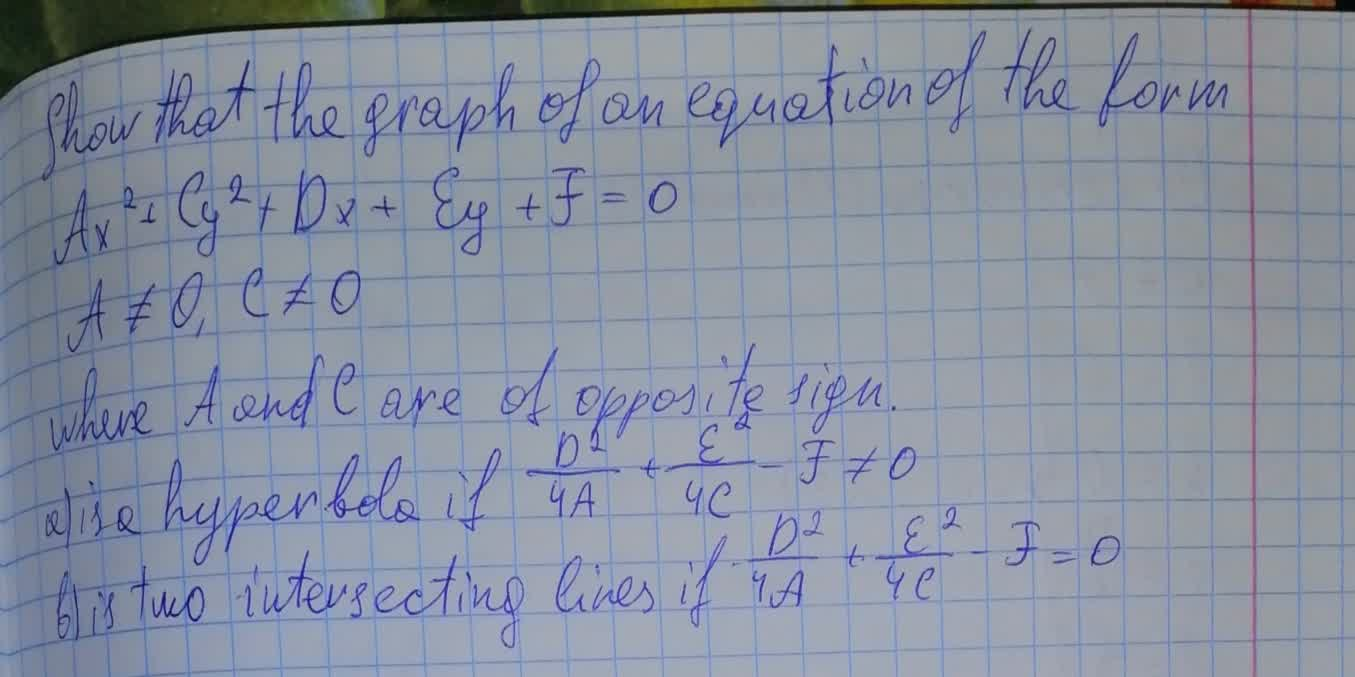Efan Halliday 2021-08-10 Answered

### Perform a rotation of axes to eliminate the xy-term, and sketch the graph of the “degenerate” conic.${x}^{2}+2xy+{y}^{2}-1=0$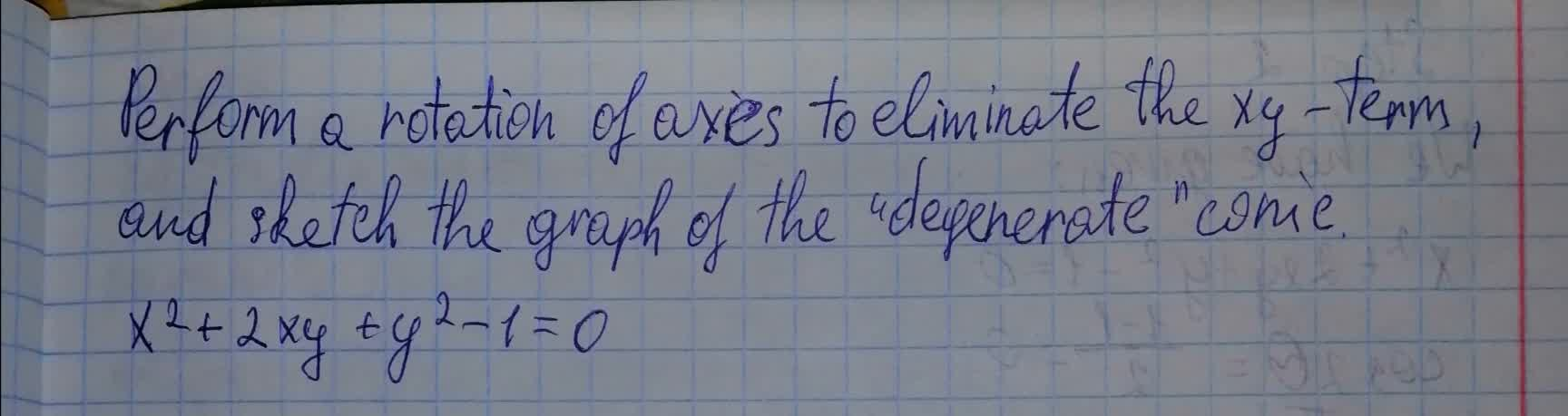nitraiddQ 2021-08-10 Answered

### Let A be a symmetric $2×2$ matrix and let k be a scalar. Prove that the graph of the quadratic equation ${x}^{T}$ Ax=k is ,an ellipse, circle, or imaginary conic if $k\ne q0$ and det A > 0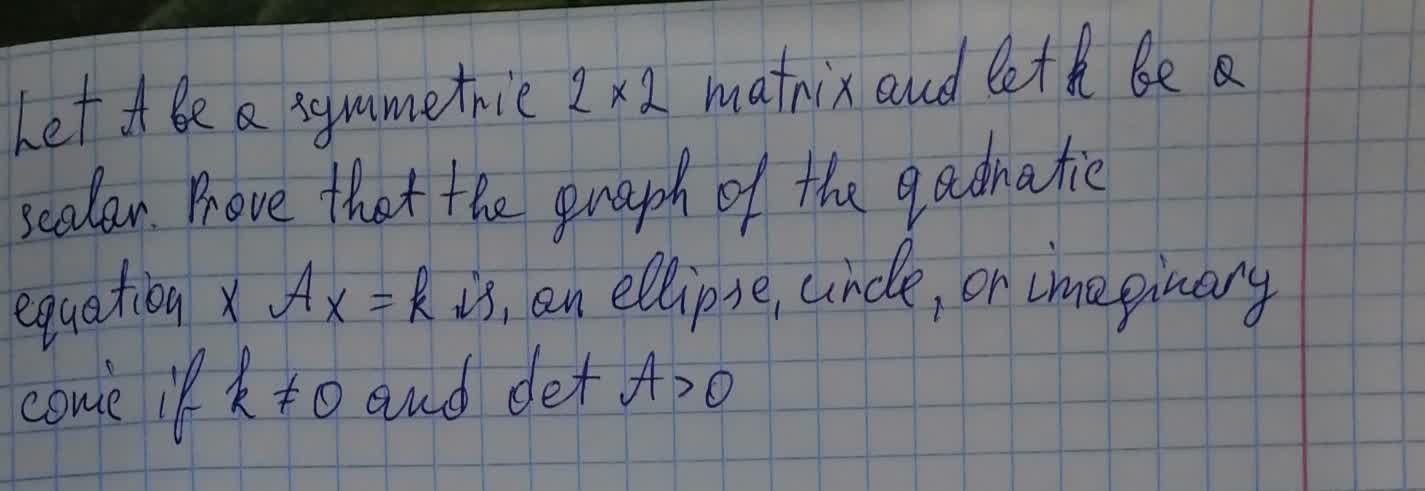nagasenaz 2021-08-10 Answered

### Find the equation of the graph for each conic in general form. Identify the conic, the center, the vertex, the co-vertex, the focus (foci), major axis, minor axis, ${a}^{2}$, ${b}^{2}$, and ${c}^{2}$. For hyperbola,find the asymtotes.Sketch the graph $x+2{y}^{2}=4$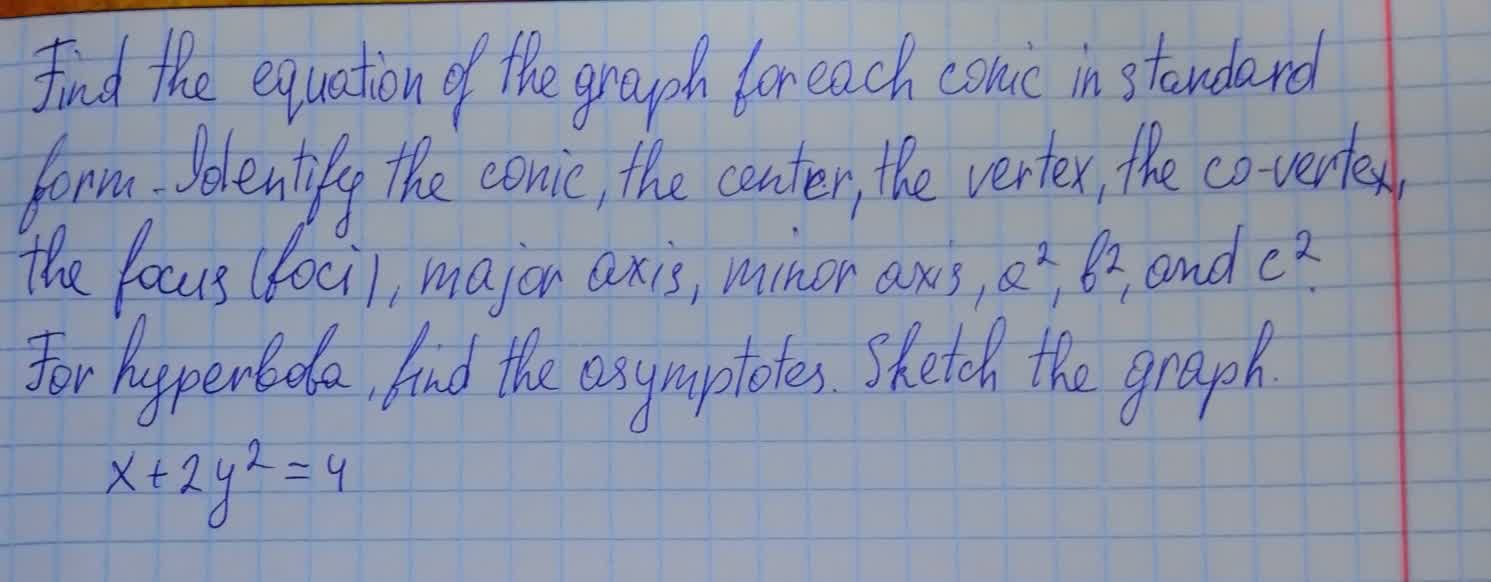ossidianaZ 2021-08-10 Answered

### Find the equation of the graph for each conic in general form. Identify the conic, the center, the vertex, the co-vertex, the focus (foci), major axis, minor axis, ${a}^{2}$, ${b}^{2}$, and ${c}^{2}$. For hyperbola,find the asymtotes.Sketch the graph $2{\left(y+2\right)}^{2}+4{\left(x-4\right)}^{2}=8$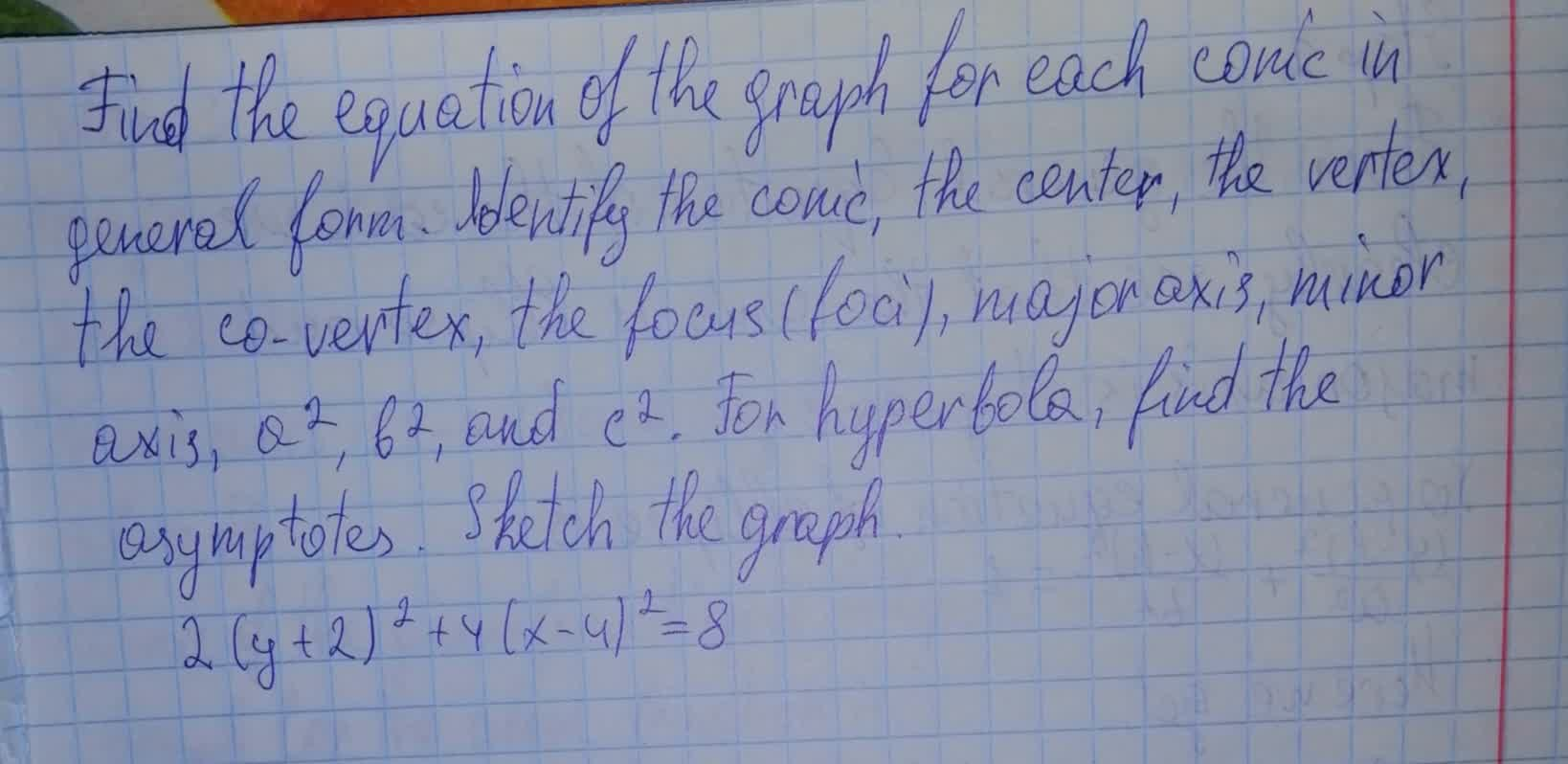Sinead Mcgee 2021-08-10 Answered

### Perform a rotation of axes to eliminate the xy-term. xy+5=0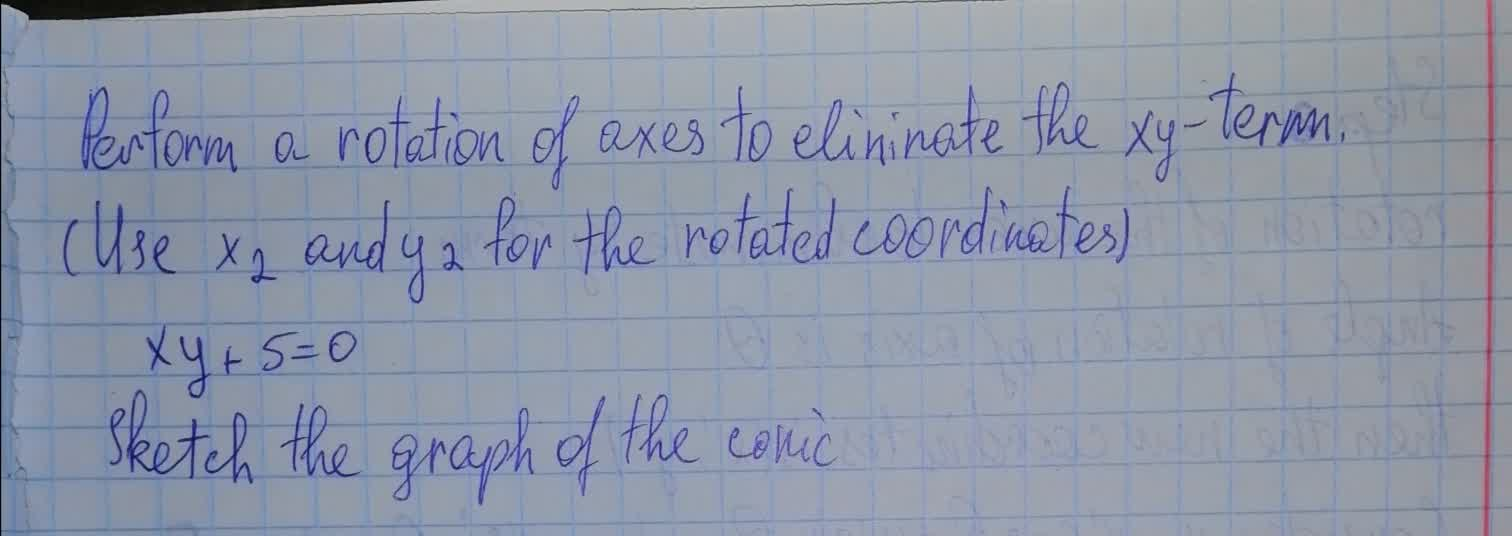preprekomW 2021-08-09 Answered

### Find the equation of the graph for each conic in standard form. Identify the conic, the center, the vertex, the co-vertex, the focus (foci), major axis, minor axis, ${a}^{2},{b}^{2}$, and ${c}^{2}$?. For hyperbola, find the asymptotes. Sketch the graph. ${y}^{2}+4y-2x+6=0$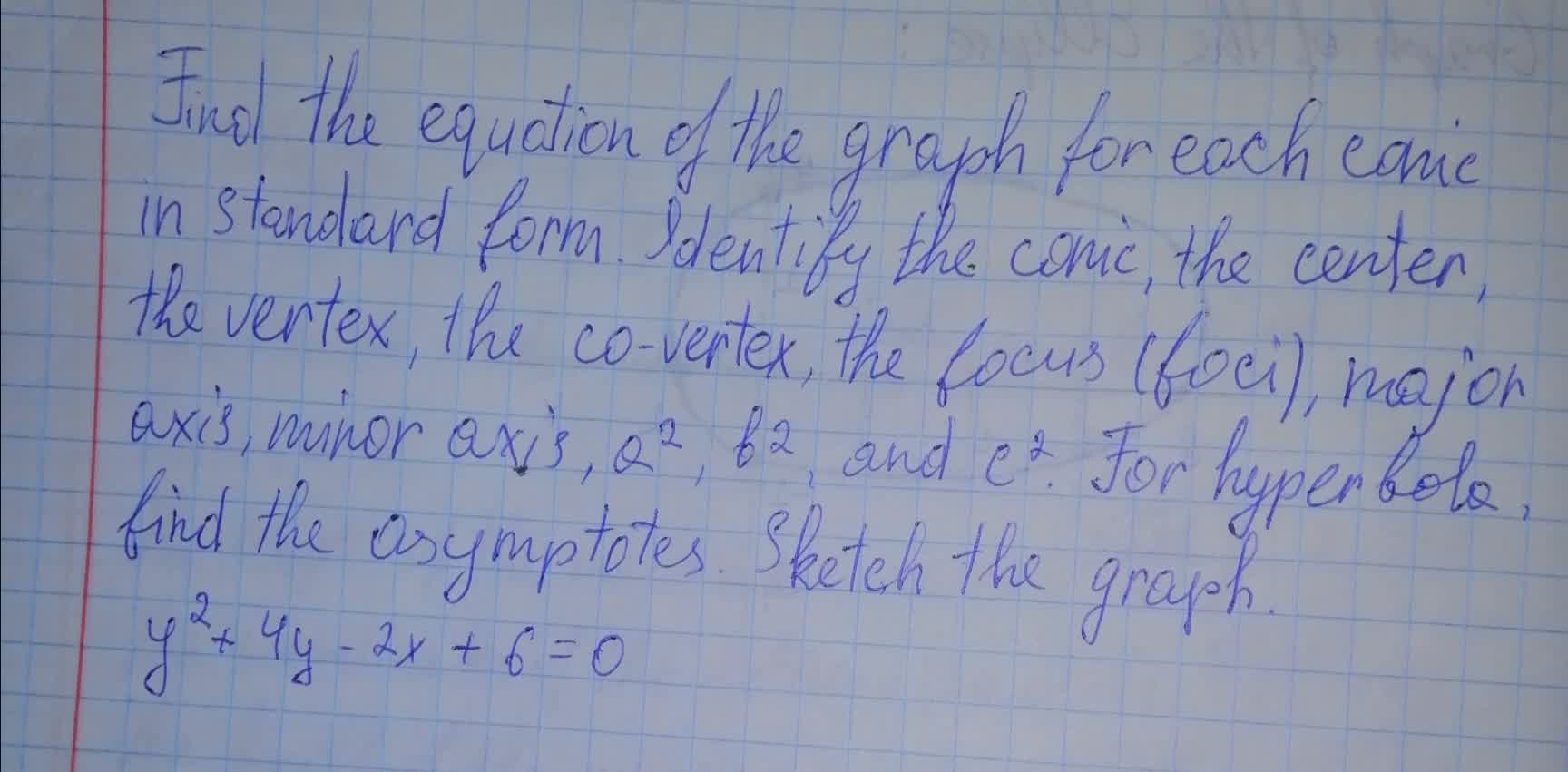kuCAu 2021-08-09 Answered

### Use the information provided to write the general conic form equation of each ellipse. $\frac{{x}^{2}}{36}+\frac{{y}^{2}}{81}=1$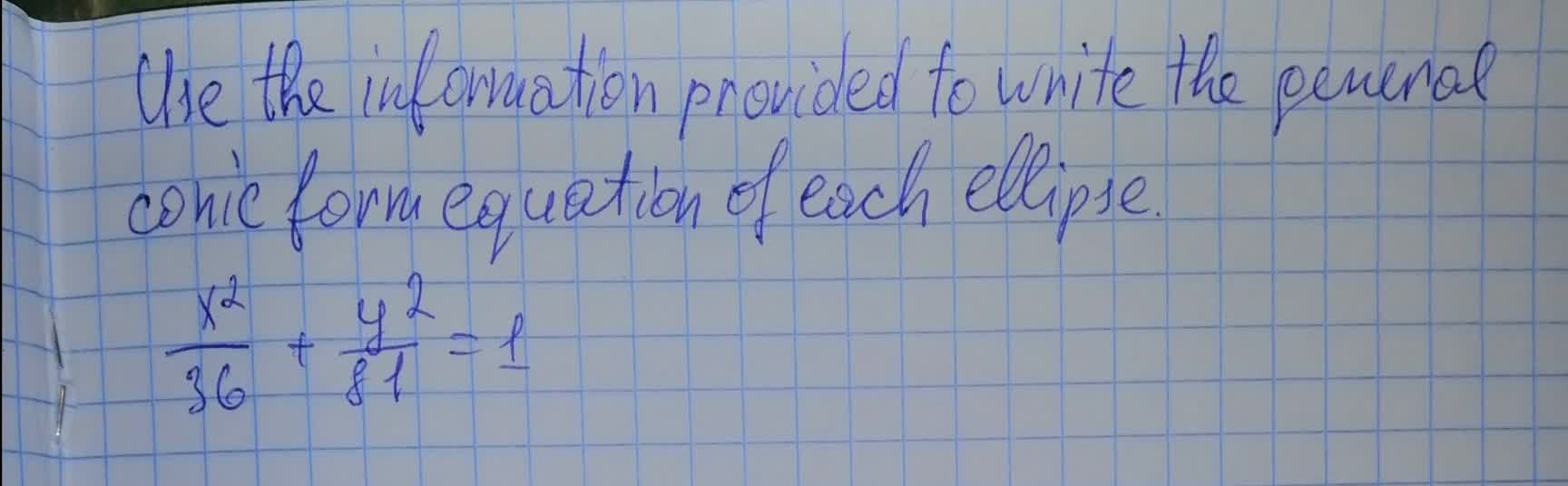bobbie71G 2021-08-09 Answered

### Show that the equation represents a conic section. Sketch the conic section, and indicate all pertinent information (such as foci, directrix, asymptotes, and so on). $\left(a\right){x}^{2}–2x–4{y}^{2}–12y=-8\left(b\right)2{x}^{2}+4x-5y+7=0\left(c\right)8{a}^{2}+8x+2{y}^{2}–20y=12$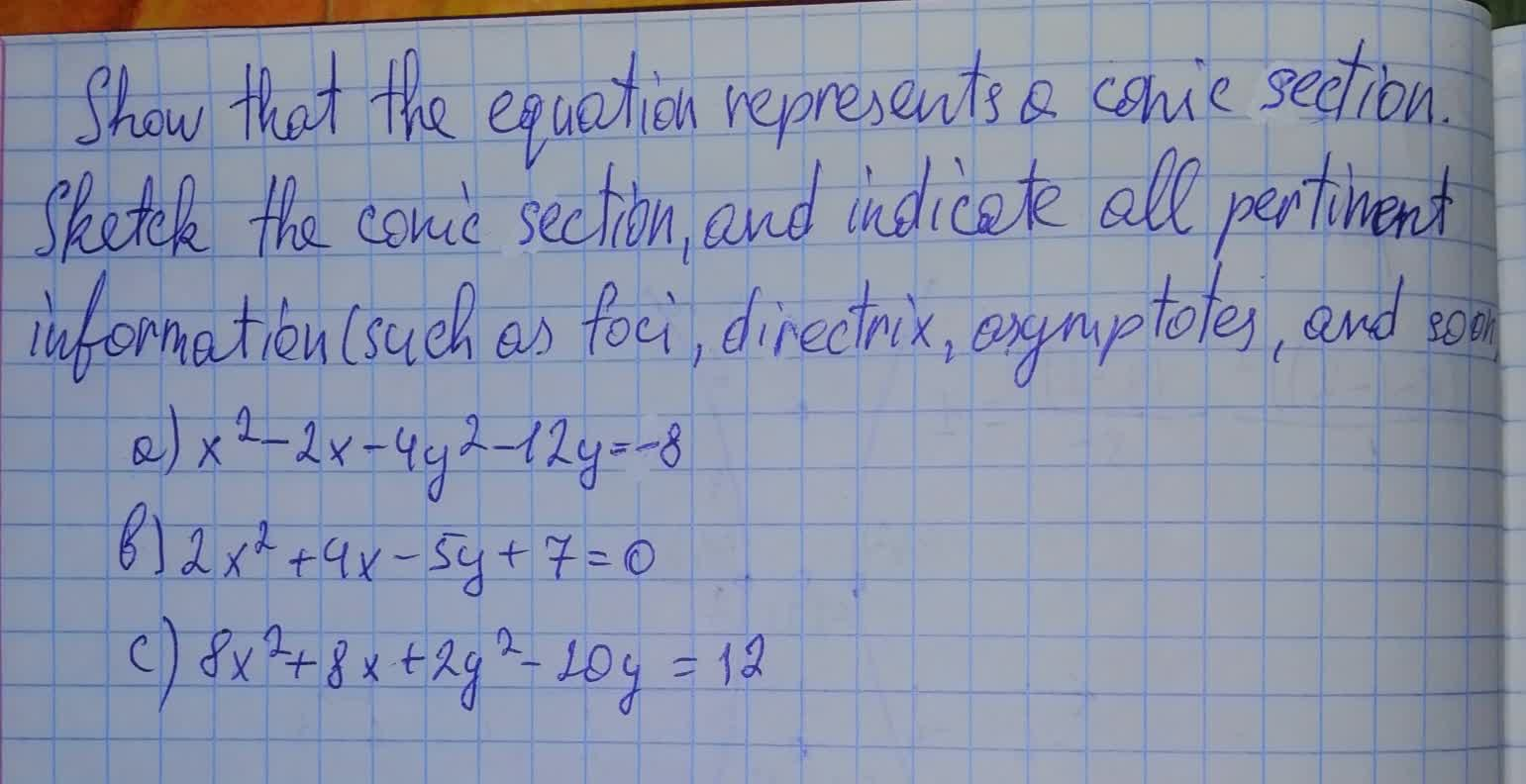floymdiT 2021-08-09 Answered

### Find the equation of the graph for each conic in general form. Identify the conic, the center, the vertex, the co-vertex, the focus (foci), major axis, minor axis, ${a}^{2}$, ${b}^{2}$, and ${c}^{2}$. For hyperbola,find the asymtotes.Sketch the graph ${\left(x-2\right)}^{2}+{\left(y-1\right)}^{2}=49$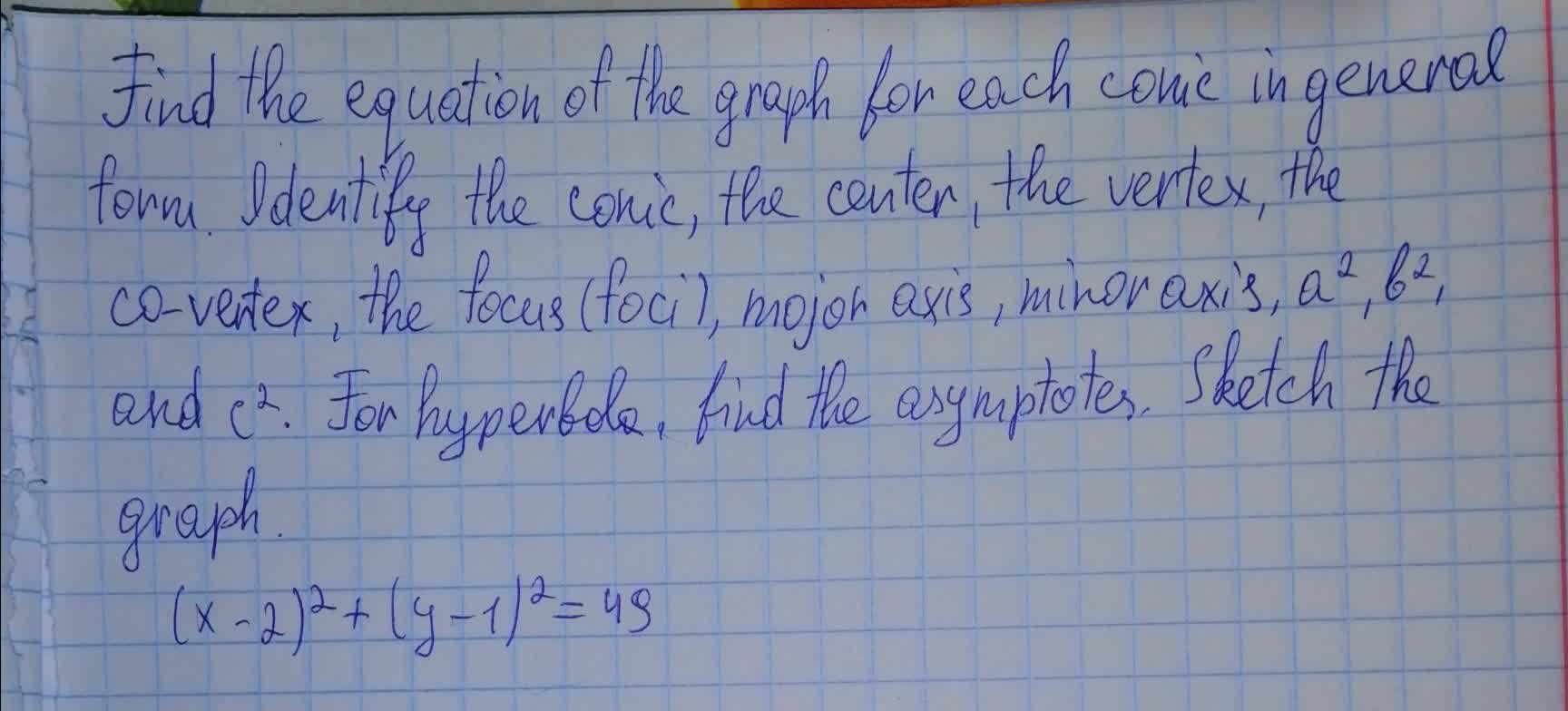lwfrgin 2021-08-08 Answered

### Find the equations of the parabolas that share a vertex and a focus with the ellipse. Draw the conics. $25{x}^{2}+9{y}^{2}=9$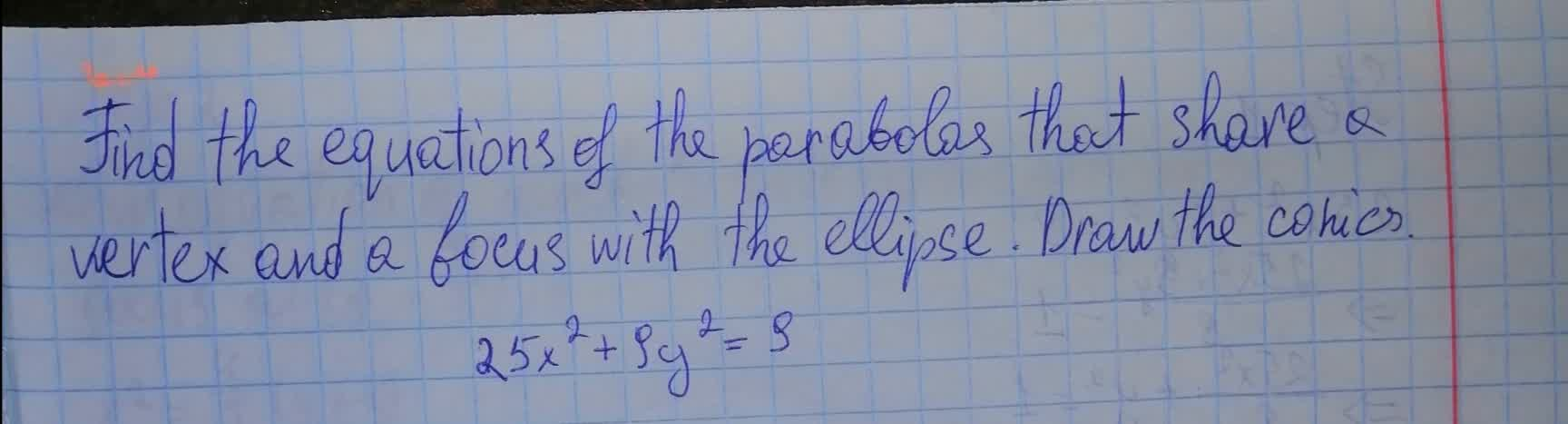usagirl007A 2021-08-08 Answered

### Draw the curve of the given equations a. ${x}^{2}+{y}^{2}+6x-12y+29=0$, b. ${y}^{2}-wx-8y+10=0$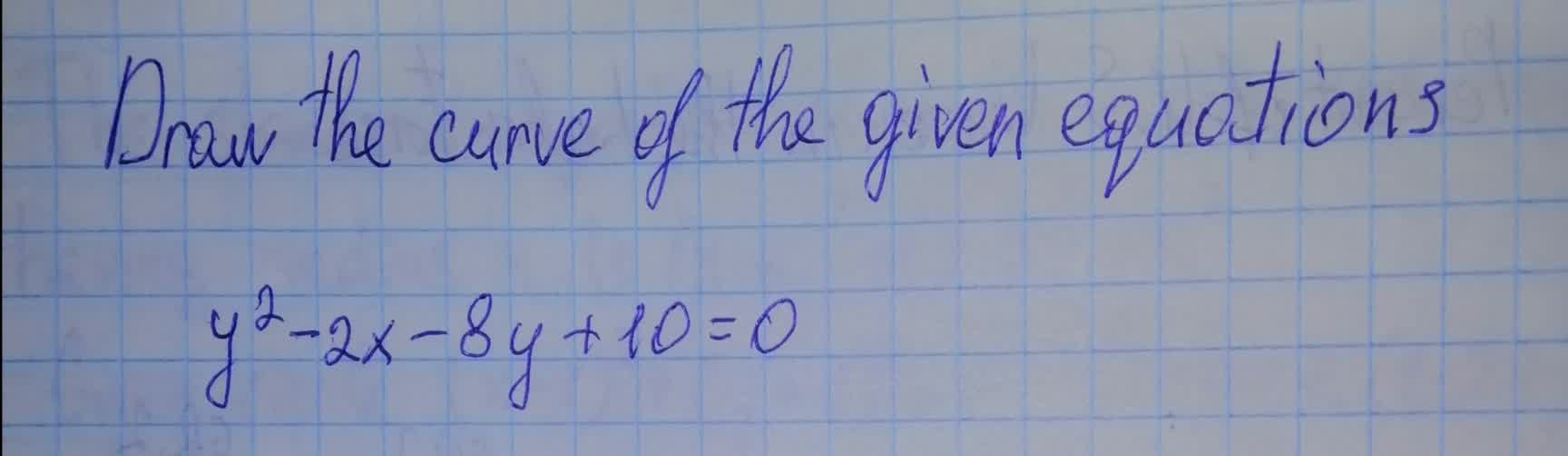Jaya Legge 2021-08-08 Answered

### Find the equation by determining the type of the conic and draw its graph. $25{x}^{2}+9{y}^{2}-100x+54y-44=0$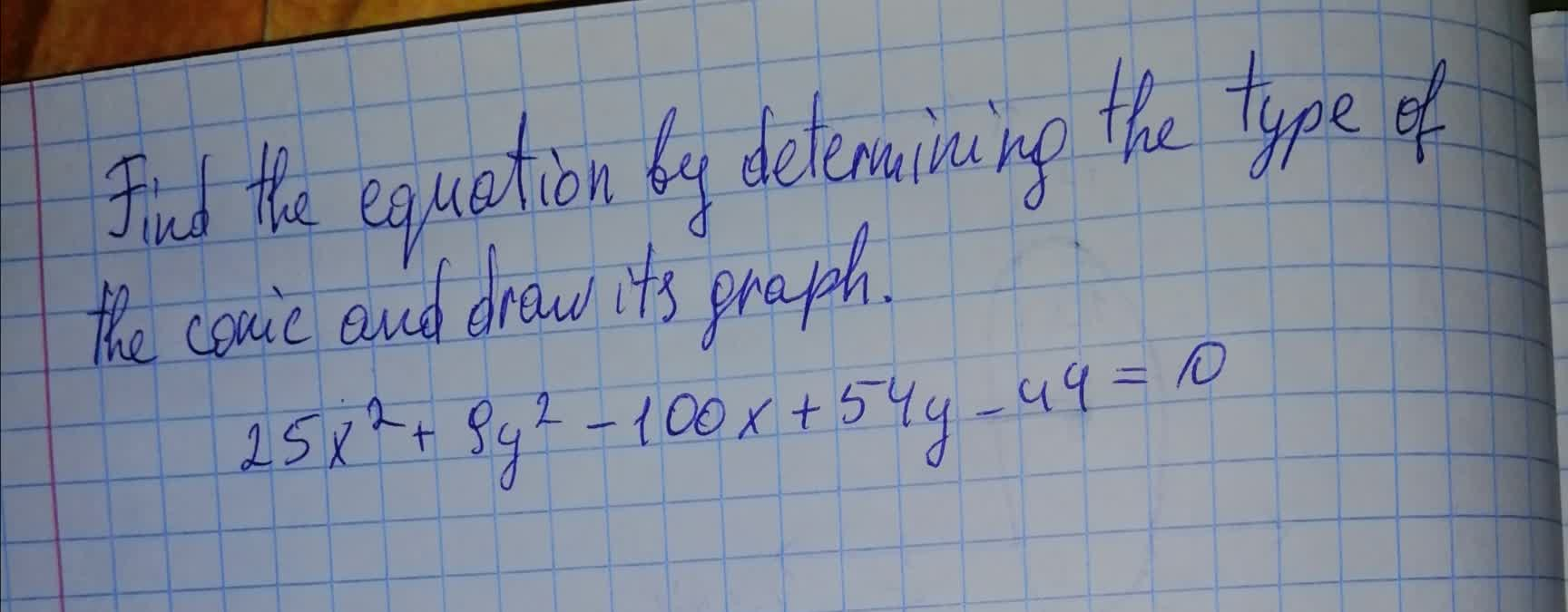BolkowN 2021-08-08 Answered

### Find an equation of the conic described.Graph the equation. Parabola; vertex at (0,0); directrix the line y=-3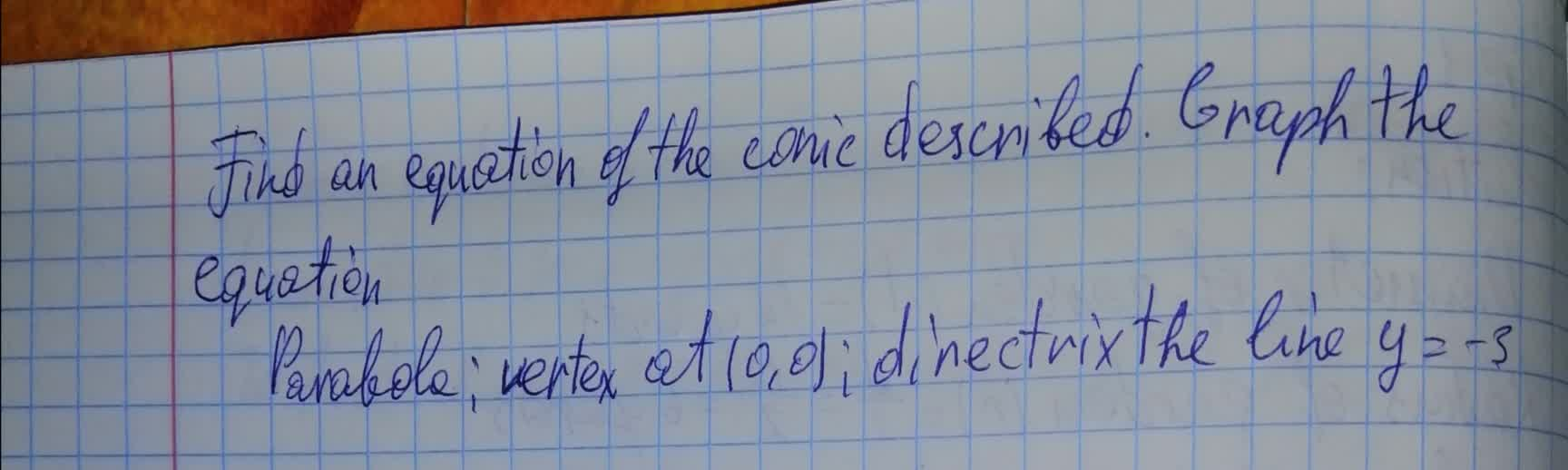Trent Carpenter 2021-08-08 Answered

### Find the equation of the graph for each conic in general form. Identify the conic, the center, the vertex, the co-vertex, the focus (foci), major axis, minor axis, ${a}^{2}$, ${b}^{2}$, and ${c}^{2}$. For hyperbola,find the asymtotes.Sketch the graph ${\left(y+7\right)}^{2}+{\left(x-5\right)}^{2}=25$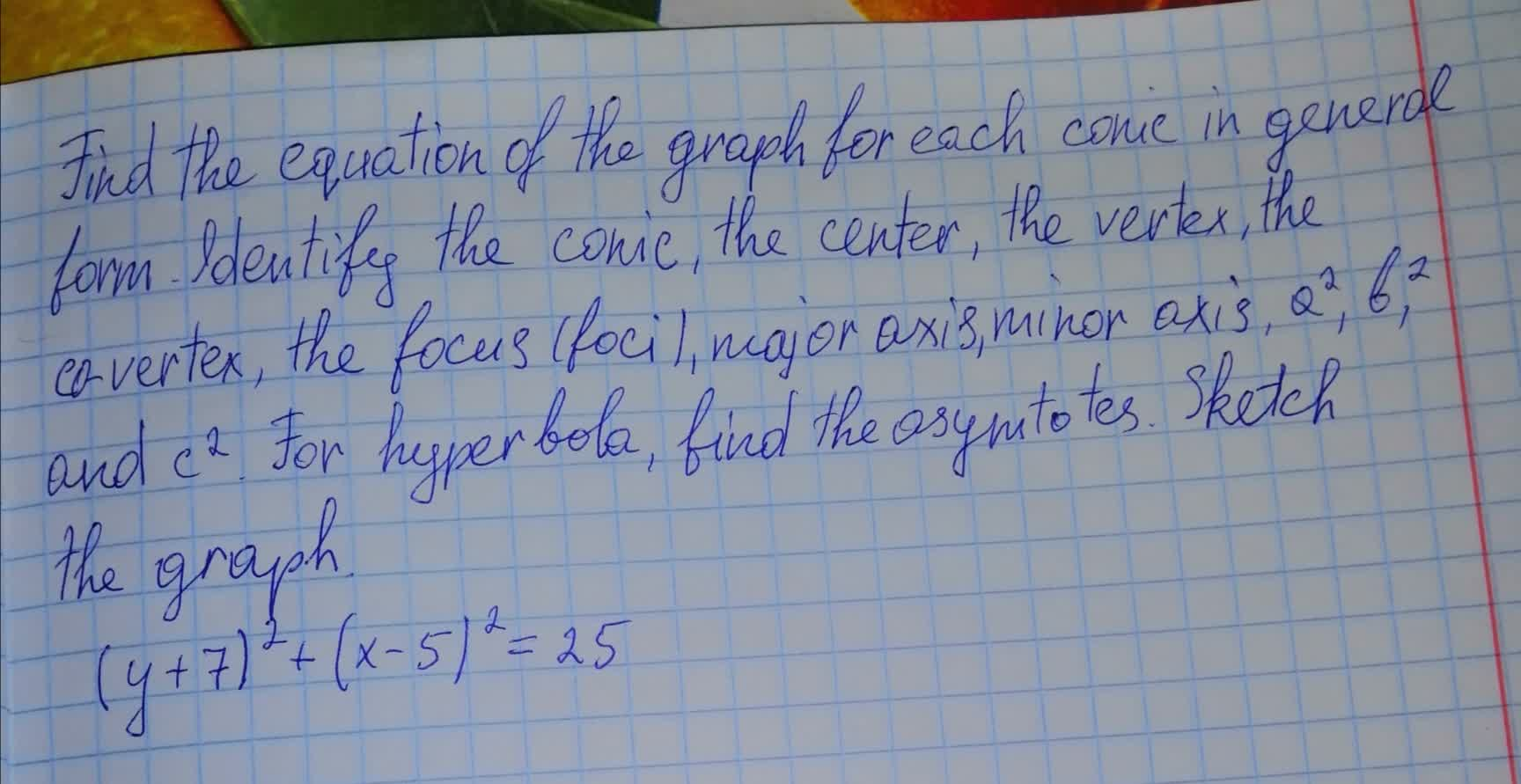texelaare 2021-08-08 Answered

### Find the equation of the graph for each conic in general form. Identify the conic, the center, the vertex, the co-vertex, the focus (foci), major axis, minor axis, ${a}^{2}$, ${b}^{2}$, and ${c}^{2}$. For hyperbola,find the asymtotes.Sketch the graph $9{\left(y-3\right)}^{2}-4{\left(x+5\right)}^{2}=36$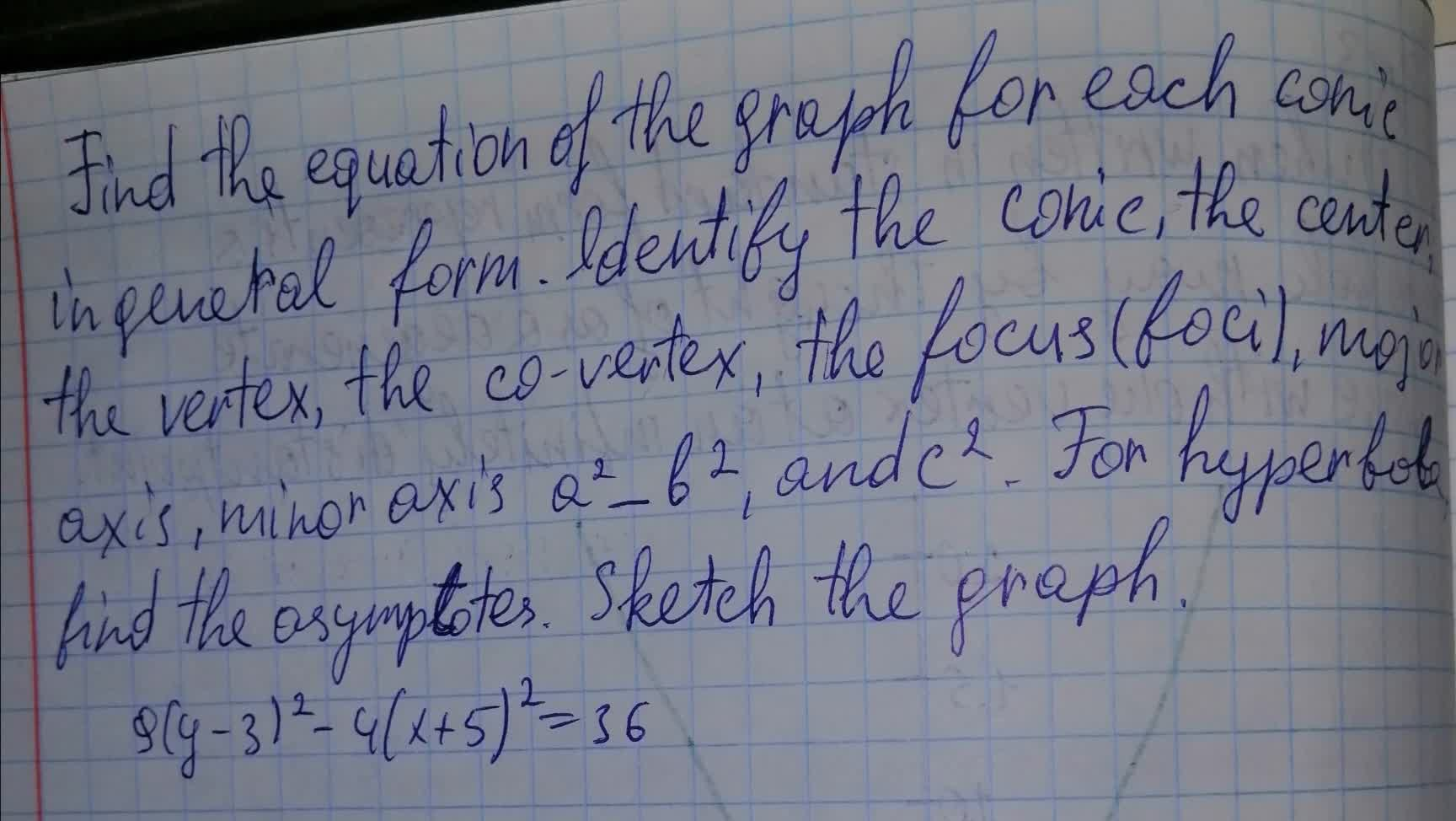ringearV 2021-08-08 Answered